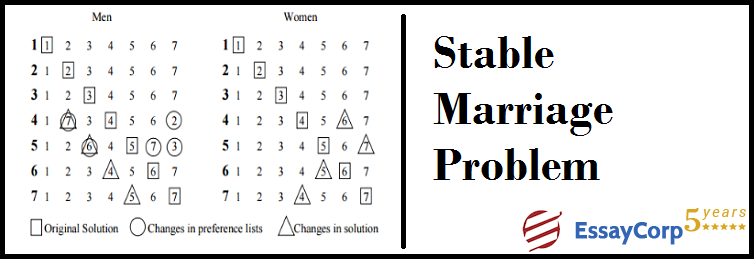The stable marriage problem is an important problem in the field of economics, mathematics and in computer science. It helps in finding the stable matching between given two sets of equal and similar type dataset with given preferences. In the given set the preference of order of dataset is given. In this problem the mapping between given set is required to be done with the following condition:

• An element in the matched set can set preferences to other matched set elements, matching can be done with other matched set too.
• The elements can prefer other matching groups too.

Accordingly to the above conditions, it is required to find the mapping between the given set. The matching can be stable only when there is no better match group then formed match.

The Stable Marriage is an important problem in the field of Computer Science.

Stable marriage problem is given as follows-

Given N men and N women, each person has ranked all the members of the opposite sex according to the order of preferences. It is required to marry the men and women in such a manner so that there are no two people of opposite sex who would both have no else partner than current partner.

Example-

Let there be two men M1 and M2 and two women W1 and W2

The preferences are as follows-

The M1 ‘s preference is –[W1,W2]

The M2’S preference is – [W1,W2]

The W1’S preference is -[M1,M2]

The W2’S preference is – [ M1,M2]

The most stable matching would be [M1,W1] and [M2,W2]

Algorithm Pseudo code-

For each women W= W1,W2, ——————Wn

S[W]= True if women is still single else false

T[W] = Temporary array or list for storing values of women who change state

R= set of list of women still single

For each Man   M= M1,M2,M3………………Mn

Step1 – Append M at the end of list R

Step2-  set min= n+1

Step3- For each pair (i, w) in Cm (change list)  set S[w]= true

T[W]= True

If i < min

w(m)= w

and   min=i

Step4- For all the women in the list at the beginning of the list R

If S[W]= False

Then        Delete w from   R, continue

If T[W]= False

And if w< min

W(m) = W

And for each pair (i , w) in Cm

T[W]= False

S[W(M)]= False

EndStable Marriage Problem  solution in c++

#include <iostream>

#include <string.h>

#include <stdio.h>

using namespace std;

// Number of Men or Women

#define N  4

// The function for defining the Man and Woman

// The following  function returns true if woman ‘w’ prefers man ‘m1’ over man ‘m’

bool W prefer M1 over M  (int prefer [2*n][n], int w, int m, int m1)

{

//function for  checking  whether w prefers m for her current engagement m1

for (int i = 0; i < n; i++)

{

// If m1 comes before m in list of w(womens), then w prefers him

// the   current engagement, don’t do anything

If  (prefer[w][i] == m1)

return true;

// If m comes before m1 in w’s list, then free her from current engagement and engage with m

if (prefer[w][i] == m)

return false;

}

}

// Prints stable matching for N women  and N Man. Mans are numbered as 0 to

// N-1. Girls are numbered as N to 2N-1.

void stable Marriage (int prefer[2*n][n])

{

// This Stores partner of women. This is our output array that

stores paring information of the man and women.  The value of w Partner[i]

indicates the partner assigned to woman n+i.

The woman numbers between n and 2*n-1. The value -1

indicates that (n+i)’th woman is free//

int  w Partner[n];

//Defining an array to store the number of available men.  If m Free[i] is

false, then man ‘i’ is free, otherwise engaged.

bool mFree[n];

// Initialize all men and women as not engaged

memset(wPartner, -1, sizeof(wPartner));

memset(mFree, false, sizeof(mFree));

int freeCount = n;

// While there are free men

while (freeCount > 0)

{

// Pick the first free man (here picking  randomly)

int m;

for (m = 0; m < n; m++)

if (mFree[m] == false)

break;

// One by one go to all women according to m’s preferences.

// Here m is the picked free man

for (int i = 0; i < n && mFree[m] == false; i++)

{

int w = prefer[m][i];

// The woman with preference is free, w and m become

partners (it is important that the partnership maybe changed

// later). So we can say they are engaged not married

if (wPartner[w-n] == -1)

{

wPartner[w-n] = m;

mFree[m] = true;

freeCount–;

}

else  // If w is not free

{

// Find current engagement of w

int m1 = wPartner[w-n];

// If w prefers m over her current engagement m1,

// then break the engagement between w and m1 and

// engage m with w.

if (wPrefersM1OverM(prefer, w, m, m1) == false)

{

wPartner[w-n] = m;

mFree[m] = true;

mFree[m1] = false;

}

} // End of Else

} // End of the for loop that goes to all women in m’s list

} // End of main while loop

// Print the solution

cout << “Woman   Man” << endl;

for (int i = 0; i < n; i++)

cout << ” ” << i+n << “\t” << wPartner[i] << endl;

}

// Driver program to test above functions

int main()

{

int prefer[2*N][N] = { {7, 5, 6, 4},

{5, 4, 6, 7},

{4, 5, 6, 7},

{4, 5, 6, 7},

{0, 1, 2, 3},

{0, 1, 2, 3},

{0, 1, 2, 3},

{0, 1, 2, 3},

};

stableMarriage(prefer);

return 0;

}Author:
Category :
Publish Date :
Social Sharing